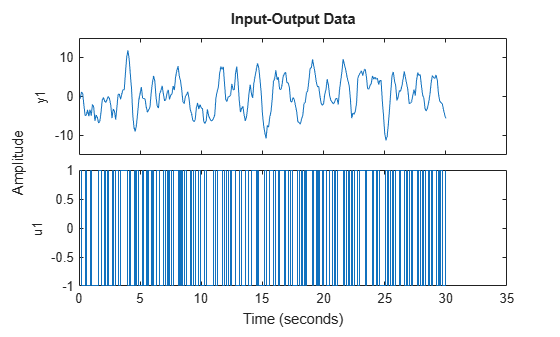# fft

Fast Fourier transform (FFT) of `iddata` object

## Syntax

``datf = fft(data)``
``datf = fft(data,N)``
``datf = fft(data,N,'complex')``

## Description

example

````datf = fft(data)` uses the fast Fourier transform (FFT) algorithm to transform the time-domain `iddata` object data to the frequency domain `iddata` object `datf`. `data` contains real-valued signals and has constant sample time `Ts`, and `datf` has frequency values equally distributed from 0 to the Nyquist frequency. The Nyquist frequency is included if the signal length is even and not included if the length is odd. To preserve the signal power and noise level, FFTs are normalized by dividing each transform by the square root of the signal length.```

example

``` `datf = fft(data,N)` specifies the transformation length `N`. In the default case, the length of the transformation is determined by the signal length. Including `N` forces the FFT transformations to pad with zeros if the signals in `data` are shorter than `N` and truncate the signals otherwise. Thus, the number of frequencies in the real signal case is `(N/2)+1` when `N` is even or `(N+1)/2` when `N` is odd. If `data` contains several experiments, `N` can be a row vector of corresponding length.```

example

``` `datf = fft(data,N,'complex')` specifies to include negative frequencies. For real signals, the default is that `datf` contains only nonnegative frequencies, while for complex-valued signals, negative frequencies are always included. To enforce negative frequencies in the real case, add `'complex'`.```

## Examples

collapse all

Load and plot the time-domain data `z1`, which contains 300 samples.

```load iddata1 z1; plot(z1)```Transform data to the frequency domain and plot.

```datf = fft(z1); plot(datf)```Load the data. Use `fft` to transform the time-domain data `z1` to the frequency domain, using a transformation length `N` of `100`.

```load iddata1 z1; datf = fft(z1, 100); plot(datf)```Load the data. Create `2` frequency-domain objects `zf1` and `zf2`, using `'complex'` when creating `zf2` to include negative frequencies.

Compare the results.

```load iddata1 z1 zf1 = fft(z1,300); zf2 = fft(z1,300,'complex'); h = plot(zf2,zf1); legend('zf2','zf1') opt = getoptions(h); opt.FreqScale = 'linear'; opt.PhaseMatching = 'on'; setoptions(h,opt) xlim([-32 32])```## Input Arguments

collapse all

Time-domain data, specified as an `iddata` object.

Transformation length, specified as a scalar integer, or for multiexperiment data, a row vector of integers. By default, each element of `N` is equal to the corresponding signal length.

## Output Arguments

collapse all

Frequency-domain data, returned as an `iddata` object.

## Tips

`fft` does not produce the same answer as the base MATLAB® `fft` function.

• For real signals, by default, `fft` computes the FFT for only the positive portion of frequency range. MATLAB `fft` computes the FFT for the entire frequency range.

• `fft` scales the result by `1/sqrt(N)`, where `N` is the data length. MATLAB `fft` does not apply scaling.

## Version History

Introduced in R2007a